1800-1023-196

+91-120-4616500

# Molecular and Ionic Equations

## Molecular and Ionic Equations

(1) Molecular equations: When the reactants and products involved in a chemical change are written in molecular forms in the chemical equation, it is termed as molecular equation.

Example: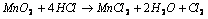In above example the reactants and products have been written in molecular forms, thus the equation is termed as molecular equation.

(2) Ionic equations: When the reactants and products involved in a chemical change are ionic compounds, these will be present in the form of ions in the solution. The chemical change is written in ionic forms in chemical equation, it is termed as ionic equation. Example,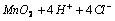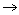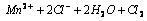In above example the reactants and products have been written in ionic forms, thus the equation is termed as ionic equation.

(3) Spectator ions: In ionic equations, the ions which do not undergo any change and equal in number in both reactants and products are termed as spectator ions and are not included in the final balanced equations. Example,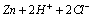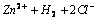(Ionic equation)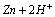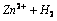(Final ionic equation)

In above example, the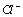ions are the spectator ions and hence are not included in the final ionic balanced equation.

## NEET & AIIMS Exam Sample Papers

 AIIMS SAMPLE PAPERS View More NEET SAMPLE PAPERS View More